|

# 如何让马桶区清爽卫生?

之前有写过卫生间的大小划分以及改善洗面区的收纳这些事，今天就讲讲小卫生间的第二部分马桶区，如何实现马桶区清爽卫生无异味。

马桶区常见的问题有：

异味难以清除；

马桶底座等边角处难打扫且易起霉；

原因在哪？

先说第一条：异味难以清除；

异味难以消除的主要原因在于马桶使用一段时间后就会在釉面沉积污垢，即使是自来水的水碱也会让原本光滑的釉面损伤，进而容易沉积，自然异味难消。

马桶区几乎所有物品（如：手指及手纸卷、废纸篓、马桶刷及刷盒）均在干湿分界线（高度1000mm）下。下部整体来说比较潮湿，物品放置或收纳于此，很容易受潮、生霉、污染，这也是难打扫的源点所在。

解决办法：

净水系统的配合及马桶的选择

净水系统中前置可解决自来水中的铁锈、泥沙等、软水机可综合水中的碱性物质；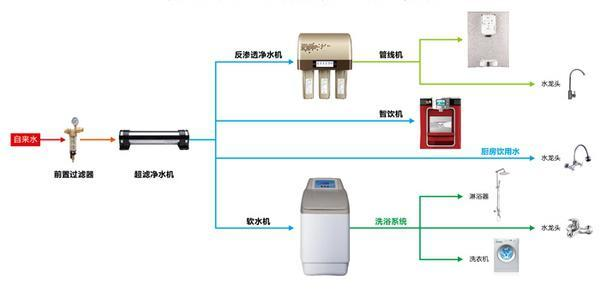马桶的选择:TOTO 的「智洁」技术利用创新的釉药，可以拥有纳米级别的超平滑表面，不给污垢容身的空隙，让他们难以黏附。不必使用过多的水和洁厕灵，让日常清洁变得轻松，减少二次污染，持久洁净。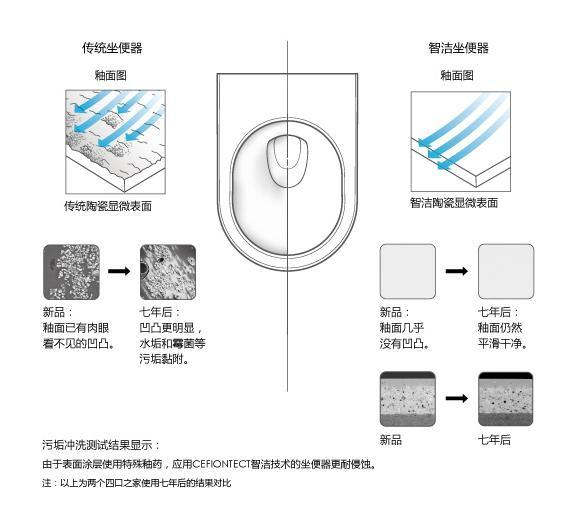节水&不易堵塞：无论你是否是环保人士，能用更少的水冲走污物都值得提倡，至少可以减少资源浪费。大小水量选择已经是今天马桶的标配，利用简单的机制即可节省能源。但每次 5.5L 以上的用水量还是不小的浪费，不少厂商一直探索利用更加先进的技术，减少用水。TOTO 的超级节水，超漩冲洗技术，科勒的五级旋风技术，不仅有一个高大上的名字，更能在冲水原理的革新基础上，实打实地起到作用。科勒，K-3834T-S 分体五级旋风马桶

地排式，最小坑距 305mm

最小用水量 4.2L

包含五级旋风技术，加长型

参考价格：￥1550（包括缓降马桶盖）

以上是未装修情况下的解决方案；在装修完毕投入使用后有这些情况的，先检查下下水管道，如没有问题却味道依旧，可尝试下“DUCK 马桶开花”这件神器。

它一管六朵，一朵冲100次左右。打出来的凝胶粘在马桶内壁，每次冲水时都能起到清洁和清香的作用。不过最好打在水量适中的地方，水量太多冲不到次数就冲掉了，水量太少则起不到作用。就是此物，参考价：￥35（马云家）

这个插座式空间消毒器会发射UV-C光（紫外线的一种），杀菌效率高达98%，可消除过敏原、异味。不光厕所，抽烟、做饭、霉变、宠物、婴儿尿布等各种味道都能消除；而且很小巧，也不耗电。不过需要使用插座变压器（60W），UV-C光灯泡7个月左右要更换。

Germ Guardian Technologies GG1000 插座式空间消毒器，参考价格：美国亚马逊售价31.96美元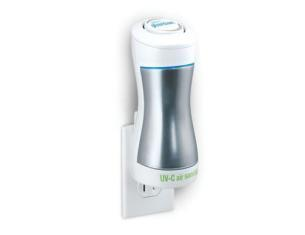Pig Kayari创意盘香座，参考价格：淘宝代购约200元

日本Reac公司设计，造型萌萌哒。陶瓷工艺，能防少量溅水；有盖子，能减少烟尘量。内部配备两种支架，盘香、线香都能使用，除了清新味道，还有提神醒脑的作用。第二条：马桶底座等边角处难打扫且易起霉；

悬挂收纳是解决此处最好的办法，如马桶可以悬挂起来，与地面没有接触，拖把可以轻松探入底部、完全没有死角；

墙排改造请参考：（挂墙式马桶链接）

此处要注意：

挂墙式马桶需要后墙排水，要在土建或装修阶段预留条件方可实施。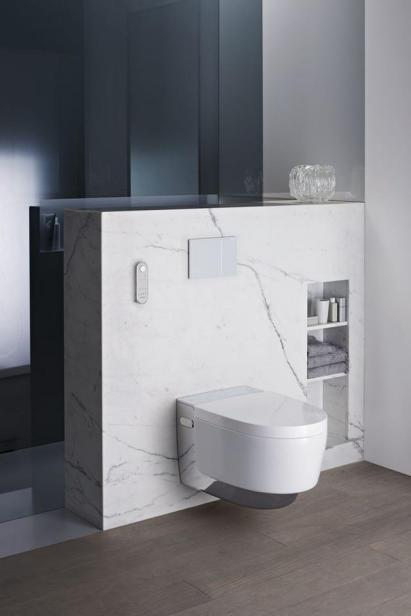关于马桶清洗，传统一直是用马桶刷，其实这样东西完全可以取消，因为马桶刷一直存在着二次污染、

细菌滋生等问题，用消毒水也只是治标不治本。

马桶刷可用“马桶喷枪”代替，这种喷枪原理是给出水加压，喷出高压水流不仅可以冲洗马桶，还可以冲洗马桶的周边区域(如:马桶背后）。

喷枪改造很简单：将原有马桶入水的三通进行更换就好，喷枪价格低廉，用知名品牌也就200左右，此点对入住和未装修的都适用。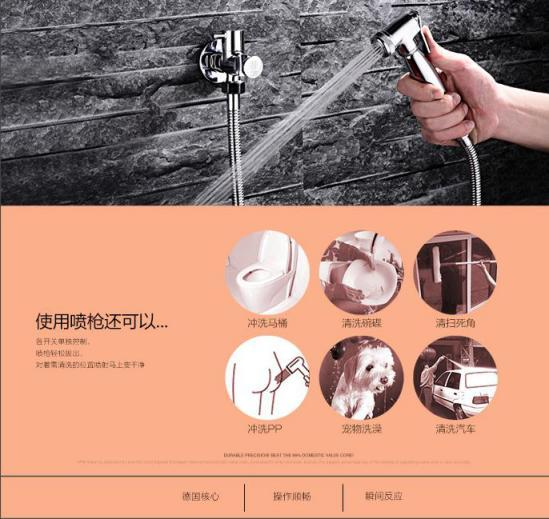垃圾桶也被诟病已久，和细菌滋生、异味也不无关系，此物也可取消，怕马桶堵的人也不用着急，虹吸马桶的确易堵，但可以对纸进行升级，用水溶性卫生纸，直接置入，遇水即溶。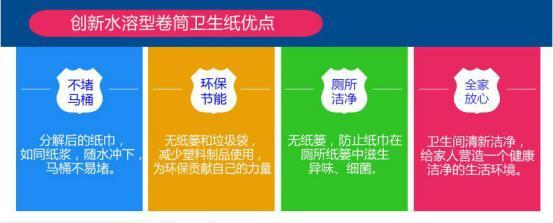对于女性清洁用品，可临时准备个袋子，用过既弃，干净卫生。

此刻，马桶区皆挂墙而置，清洁起来自然倍感轻松。

最后，在这里手动打个广告，住好家开通了微信订阅号，大家可以搜索【住好家指南】，我们有专业的设计师可以给大家提供装修建议，只要你提供需求，我们可以给您家免费进行装修方案评估和主材预算评估，并且承诺在24小时完成评估报告，有兴趣的可以添加我们的公众号哦~~

（本文为住好家原创，欢迎转发分享）

声明：近期文章推荐主材选择，涉及到一些品牌，只是根据网友真实反馈进行说明，所述仅代表【住好家】品牌设计师和家居编辑个人观点，绝对不存在给任何品牌做广告的嫌疑

`声明：本文由入驻焦点开放平台的作者撰写，除焦点官方账号外，观点仅代表作者本人，不代表焦点立场错误信息举报电话： 400-099-0099，邮箱：jubao@vip.sohu.com，或点此进行意见反馈，或点此进行举报投诉。`A B C D E F G H J K L M N P Q R S T W X Y Z
A - B - C - D - E
• A
• 鞍山
• 安庆
• 安阳
• 安顺
• 安康
• 澳门
• B
• 北京
• 保定
• 包头
• 巴彦淖尔
• 本溪
• 蚌埠
• 亳州
• 滨州
• 北海
• 百色
• 巴中
• 毕节
• 保山
• 宝鸡
• 白银
• 巴州
• C
• 承德
• 沧州
• 长治
• 赤峰
• 朝阳
• 长春
• 常州
• 滁州
• 池州
• 长沙
• 常德
• 郴州
• 潮州
• 崇左
• 重庆
• 成都
• 楚雄
• 昌都
• 慈溪
• 常熟
• D
• 大同
• 大连
• 丹东
• 大庆
• 东营
• 德州
• 东莞
• 德阳
• 达州
• 大理
• 德宏
• 定西
• 儋州
• 东平
• E
• 鄂尔多斯
• 鄂州
• 恩施
F - G - H - I - J
• F
• 抚顺
• 阜新
• 阜阳
• 福州
• 抚州
• 佛山
• 防城港
• G
• 赣州
• 广州
• 桂林
• 贵港
• 广元
• 广安
• 贵阳
• 固原
• H
• 邯郸
• 衡水
• 呼和浩特
• 呼伦贝尔
• 葫芦岛
• 哈尔滨
• 黑河
• 淮安
• 杭州
• 湖州
• 合肥
• 淮南
• 淮北
• 黄山
• 菏泽
• 鹤壁
• 黄石
• 黄冈
• 衡阳
• 怀化
• 惠州
• 河源
• 贺州
• 河池
• 海口
• 红河
• 汉中
• 海东
• I
• J
• 晋中
• 锦州
• 吉林
• 鸡西
• 佳木斯
• 嘉兴
• 金华
• 景德镇
• 九江
• 吉安
• 济南
• 济宁
• 焦作
• 荆门
• 荆州
• 江门
• 揭阳
• 金昌
• 酒泉
• 嘉峪关
K - L - M - N - P
• K
• 开封
• 昆明
• 昆山
• L
• 廊坊
• 临汾
• 辽阳
• 连云港
• 丽水
• 六安
• 龙岩
• 莱芜
• 临沂
• 聊城
• 洛阳
• 漯河
• 娄底
• 柳州
• 来宾
• 泸州
• 乐山
• 六盘水
• 丽江
• 临沧
• 拉萨
• 林芝
• 兰州
• 陇南
• M
• 牡丹江
• 马鞍山
• 茂名
• 梅州
• 绵阳
• 眉山
• N
• 南京
• 南通
• 宁波
• 南平
• 宁德
• 南昌
• 南阳
• 南宁
• 内江
• 南充
• P
• 盘锦
• 莆田
• 平顶山
• 濮阳
• 攀枝花
• 普洱
• 平凉
Q - R - S - T - W
• Q
• 秦皇岛
• 齐齐哈尔
• 衢州
• 泉州
• 青岛
• 清远
• 钦州
• 黔南
• 曲靖
• 庆阳
• R
• 日照
• 日喀则
• S
• 石家庄
• 沈阳
• 双鸭山
• 绥化
• 上海
• 苏州
• 宿迁
• 绍兴
• 宿州
• 三明
• 上饶
• 三门峡
• 商丘
• 十堰
• 随州
• 邵阳
• 韶关
• 深圳
• 汕头
• 汕尾
• 三亚
• 三沙
• 遂宁
• 山南
• 商洛
• 石嘴山
• T
• 天津
• 唐山
• 太原
• 通辽
• 铁岭
• 泰州
• 台州
• 铜陵
• 泰安
• 铜仁
• 铜川
• 天水
• 天门
• W
• 乌海
• 乌兰察布
• 无锡
• 温州
• 芜湖
• 潍坊
• 威海
• 武汉
• 梧州
• 渭南
• 武威
• 吴忠
• 乌鲁木齐
X - Y - Z
• X
• 邢台
• 徐州
• 宣城
• 厦门
• 新乡
• 许昌
• 信阳
• 襄阳
• 孝感
• 咸宁
• 湘潭
• 湘西
• 西双版纳
• 西安
• 咸阳
• 西宁
• 仙桃
• 西昌
• Y
• 运城
• 营口
• 盐城
• 扬州
• 鹰潭
• 宜春
• 烟台
• 宜昌
• 岳阳
• 益阳
• 永州
• 阳江
• 云浮
• 玉林
• 宜宾
• 雅安
• 玉溪
• 延安
• 榆林
• 银川
• Z
• 张家口
• 镇江
• 舟山
• 漳州
• 淄博
• 枣庄
• 郑州
• 周口
• 驻马店
• 株洲
• 张家界
• 珠海
• 湛江
• 肇庆
• 中山
• 自贡
• 资阳
• 遵义
• 昭通
• 张掖
• 中卫

1室1厅1厨1卫1阳台

1
2
3
4
5

0
1
2

1

1

0
1
2
3报名成功，资料已提交审核A B C D E F G H J K L M N P Q R S T W X Y Z
A - B - C - D - E
• A
• 鞍山
• 安庆
• 安阳
• 安顺
• 安康
• 澳门
• B
• 北京
• 保定
• 包头
• 巴彦淖尔
• 本溪
• 蚌埠
• 亳州
• 滨州
• 北海
• 百色
• 巴中
• 毕节
• 保山
• 宝鸡
• 白银
• 巴州
• C
• 承德
• 沧州
• 长治
• 赤峰
• 朝阳
• 长春
• 常州
• 滁州
• 池州
• 长沙
• 常德
• 郴州
• 潮州
• 崇左
• 重庆
• 成都
• 楚雄
• 昌都
• 慈溪
• 常熟
• D
• 大同
• 大连
• 丹东
• 大庆
• 东营
• 德州
• 东莞
• 德阳
• 达州
• 大理
• 德宏
• 定西
• 儋州
• 东平
• E
• 鄂尔多斯
• 鄂州
• 恩施
F - G - H - I - J
• F
• 抚顺
• 阜新
• 阜阳
• 福州
• 抚州
• 佛山
• 防城港
• G
• 赣州
• 广州
• 桂林
• 贵港
• 广元
• 广安
• 贵阳
• 固原
• H
• 邯郸
• 衡水
• 呼和浩特
• 呼伦贝尔
• 葫芦岛
• 哈尔滨
• 黑河
• 淮安
• 杭州
• 湖州
• 合肥
• 淮南
• 淮北
• 黄山
• 菏泽
• 鹤壁
• 黄石
• 黄冈
• 衡阳
• 怀化
• 惠州
• 河源
• 贺州
• 河池
• 海口
• 红河
• 汉中
• 海东
• I
• J
• 晋中
• 锦州
• 吉林
• 鸡西
• 佳木斯
• 嘉兴
• 金华
• 景德镇
• 九江
• 吉安
• 济南
• 济宁
• 焦作
• 荆门
• 荆州
• 江门
• 揭阳
• 金昌
• 酒泉
• 嘉峪关
K - L - M - N - P
• K
• 开封
• 昆明
• 昆山
• L
• 廊坊
• 临汾
• 辽阳
• 连云港
• 丽水
• 六安
• 龙岩
• 莱芜
• 临沂
• 聊城
• 洛阳
• 漯河
• 娄底
• 柳州
• 来宾
• 泸州
• 乐山
• 六盘水
• 丽江
• 临沧
• 拉萨
• 林芝
• 兰州
• 陇南
• M
• 牡丹江
• 马鞍山
• 茂名
• 梅州
• 绵阳
• 眉山
• N
• 南京
• 南通
• 宁波
• 南平
• 宁德
• 南昌
• 南阳
• 南宁
• 内江
• 南充
• P
• 盘锦
• 莆田
• 平顶山
• 濮阳
• 攀枝花
• 普洱
• 平凉
Q - R - S - T - W
• Q
• 秦皇岛
• 齐齐哈尔
• 衢州
• 泉州
• 青岛
• 清远
• 钦州
• 黔南
• 曲靖
• 庆阳
• R
• 日照
• 日喀则
• S
• 石家庄
• 沈阳
• 双鸭山
• 绥化
• 上海
• 苏州
• 宿迁
• 绍兴
• 宿州
• 三明
• 上饶
• 三门峡
• 商丘
• 十堰
• 随州
• 邵阳
• 韶关
• 深圳
• 汕头
• 汕尾
• 三亚
• 三沙
• 遂宁
• 山南
• 商洛
• 石嘴山
• T
• 天津
• 唐山
• 太原
• 通辽
• 铁岭
• 泰州
• 台州
• 铜陵
• 泰安
• 铜仁
• 铜川
• 天水
• 天门
• W
• 乌海
• 乌兰察布
• 无锡
• 温州
• 芜湖
• 潍坊
• 威海
• 武汉
• 梧州
• 渭南
• 武威
• 吴忠
• 乌鲁木齐
X - Y - Z
• X
• 邢台
• 徐州
• 宣城
• 厦门
• 新乡
• 许昌
• 信阳
• 襄阳
• 孝感
• 咸宁
• 湘潭
• 湘西
• 西双版纳
• 西安
• 咸阳
• 西宁
• 仙桃
• 西昌
• Y
• 运城
• 营口
• 盐城
• 扬州
• 鹰潭
• 宜春
• 烟台
• 宜昌
• 岳阳
• 益阳
• 永州
• 阳江
• 云浮
• 玉林
• 宜宾
• 雅安
• 玉溪
• 延安
• 榆林
• 银川
• Z
• 张家口
• 镇江
• 舟山
• 漳州
• 淄博
• 枣庄
• 郑州
• 周口
• 驻马店
• 株洲
• 张家界
• 珠海
• 湛江
• 肇庆
• 中山
• 自贡
• 资阳
• 遵义
• 昭通
• 张掖
• 中卫• 手机• 分享
• 设计
免费设计
• 计算器
装修计算器
• 入驻
合作入驻
• 联系
联系我们
• 置顶
返回顶部